# Exercise E8.2 Quadrilaterals NCERT Solutions Class 9

## Chapter 8 Ex.8.2 Question 1

$$ABCD$$ is a quadrilateral in which $$P$$, $$Q$$, $$R$$ and $$S$$ are mid-points of the sides $$AB$$, $$BC$$, $$CD$$ and $$DA$$ (see the given figure). $$AC$$ is a diagonal. Show that: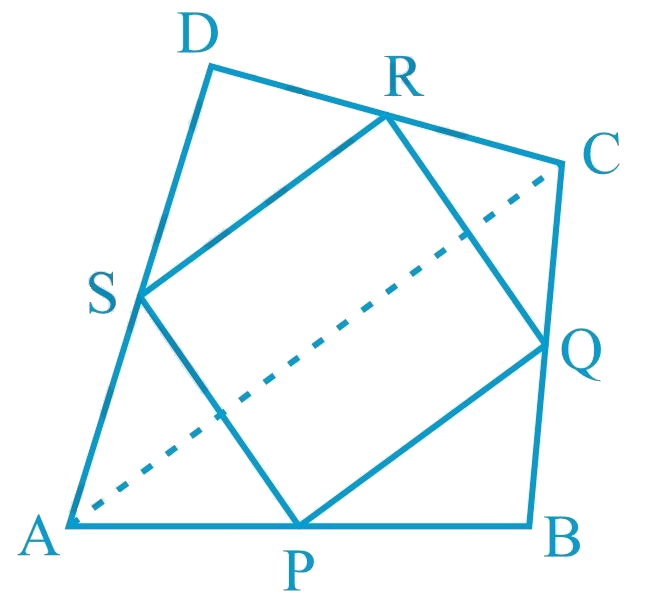i) \begin{align}{SR}\; \| \; {AC} \; \rm{and} \; {SR}=\frac{1}{2} {AC} \end{align}

ii) $$PQ = SR$$

iii) $$PQRS$$ is a parallelogram.

### Solution

What is known?

$$ABCD$$ is a quadrilateral in which $$P, Q, R$$ and $$S$$ are mid-points of the sides $$AB, BC, CD$$ and $$DA. AC$$ is a diagonal.

What is unknown?

How we can show that

(i) $$SR\; AC$$ and $$SR \;AC$$

(ii) $$PQ SR$$

(iii) $$PQRS$$ is a parallelogram.

Reasoning:

In a triangle, the line segment joining the mid-points of any two sides of the triangle is parallel to the third side and is half of it. Also, if one pair of opposite sides of quadrilateral is parallel and equal to each other then it is a parallelogram.

Steps:

(i) In $$\Delta$$$$ADC$$, $$S$$ and $$R$$ are the mid-points of sides $$AD$$ and $$CD$$ respectively. In a triangle, the line segment joining the mid-points of any two sides of the triangle is parallel to the third side and is half of it.

\begin{align}\therefore SR \parallel AC \;and \; SR\!=\!\frac{1}{2}\ AC\quad\!\!... (1)\end{align}

(ii) In $$\Delta$$$$ABC$$, $$P$$ and $$Q$$ are mid-points of sides $$AB$$ and $$BC$$ respectively. Therefore, by using mid-point theorem,

\begin{align} PQ \parallel AC\; and \ PQ \; \!=\!\!\frac{1}{2} AC\quad\!\!... (2)\end{align}

Using Equations ($$1$$) and ($$2$$), we obtain$$PQ \; \| \;SR$$ and $$PQ = SR$$.. (3)

\begin{align} \therefore \rm PQ = SR \end{align}

(iii) From Equation ($$3$$), we obtained $$PQ$$ $$\parallel$$ $$SR$$ and $$PQ =SR$$

Clearly, one pair of opposite sides of quadrilateral $$PQRS$$ is parallel and equal.

Hence, $$PQRS$$ is a parallelogram.

## Chapter 8 Ex.8.2 Question 2

$$ABCD$$ is a rhombus and $$P$$, $$Q$$, $$R$$ and $$S$$ are the mid-points of the sides $$AB$$, $$BC$$, $$CD$$ and $$DA$$ respectively. Show that the quadrilateral $$PQRS$$ is a rectangle.

### Solution

What is known?

$$ABCD$$ is a rhombus and $$P, Q, R$$ and $$S$$ are the mid-points of the sides $$AB, BC, CD$$and $$DA$$ respectively.

What is unknown?

How we can show that quadrilateral $$PQRS$$ is a rectangle.

Reasoning:

In a triangle, the line segment joining the mid-points of any two sides of the triangle is parallel to the third side and is half of it. Also if one pair of opposite sides of quadrilateral is parallel and equal to each other then it is a parallelogram. By showing one of the angle in parallelogram as $$90$$ degree then we can say it is rectangle.

Steps: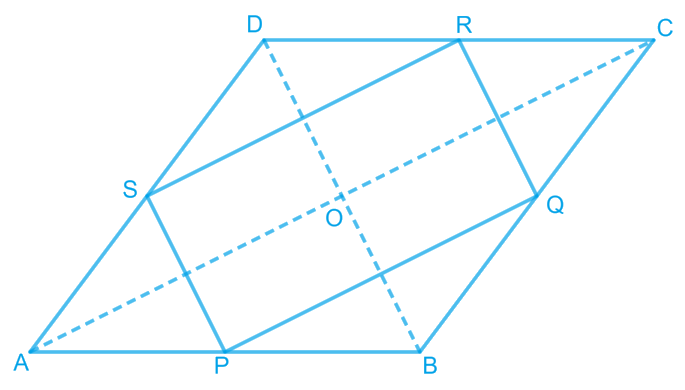In $$\rm \Delta ABC,$$ $$P$$ and $$Q$$ are the mid-points of sides $$AB$$ and $$BC$$ respectively.

\therefore \begin{align} \rm PQ \parallel AC \; and \; PQ =\frac{1}{2} \text{AC} \end{align}  (Using mid-point theorem).....(1)

In $$\Delta \text{ADC,}$$

$$R$$ and $$S$$ are the mid-points of $$CD$$ and $$AD$$ respectively.

\therefore \begin{align} RS \parallel AC \; and \; RS=\frac{1}{2} \text{AC } \end{align} (Using mid-point theorem) ....(2)

From Equations (1) and (2), we obtain

\begin{align} PQ \parallel RS \; and \; PQ= RS \end{align}

Since in quadrilateral $$PQRS$$, one pair of opposite sides is equal and parallel to each other, it is a parallelogram.

Let the diagonals of rhombus $$ABCD$$ intersect each other at point $$O$$.

In quadrilateral $$OMQN$$,

\begin{align} MQ \parallel ON \; \; ( \because PQ \parallel AC) \end{align}

\begin{align} QN \parallel OM \; \; ( \because QR \parallel BD) \end{align}

Therefore, $$OMQN$$ is a parallelogram.

\begin{align} \therefore \angle MQN = \angle NOM \end{align}

\begin{align} \therefore \angle PQR = \angle NOM \end{align}

However,\begin{align} \angle NOM = 90^\circ\end{align} (Diagonals of a rhombus are perpendicular to each other)

\begin{align} \therefore \angle PQR = 90^\circ\end{align}

Clearly, $$PQRS$$ is a parallelogram having one of its interior angles as $$90^\circ$$ .

Hence, $$PQRS$$ is a rectangle.

## Chapter 8 Ex.8.2 Question 3

$$ABCD$$ is a rectangle and $$P$$, $$Q$$, $$R$$ and $$S$$ are mid-points of the sides $$AB$$, $$BC$$, $$CD$$ and $$DA$$ respectively. Show that the quadrilateral $$PQRS$$ is a rhombus.

### Solution

What is known?

$$ABCD$$ is a rectangle and $$P, Q, R$$ and $$S$$ are the mid-points of the sides$$AB, BC, CD\,and\, DA$$ respectively.

What is unknown?

How we can show that quadrilateral $$PQRS$$ is a rhombus.

Reasoning:

In a triangle, the line segment joining the mid-points of any two sides of the triangle is parallel to the third side and is half of it. Also if one pair of opposite sides of quadrilateral is parallel and equal to each other then it is a parallelogram. By showing all four sides equal in parallelogram we can say it is rhombus.

Steps: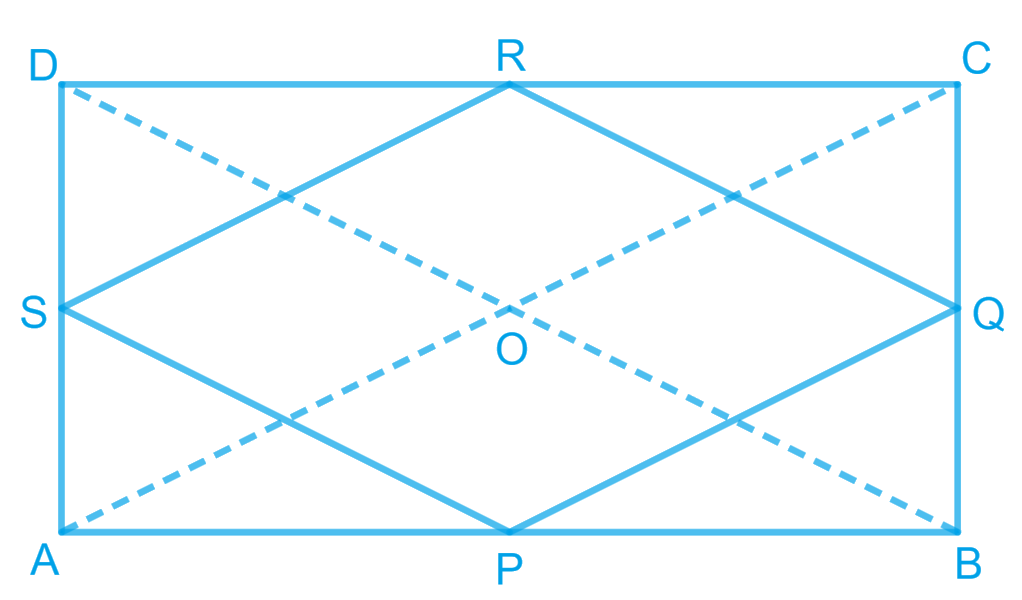Let us join $$AC$$ and $$BD$$.

In $$\Delta$$$$ABC$$,

$$P$$ and $$Q$$ are the mid-points of $$AB$$ and $$BC$$ respectively.

\begin{align}&\therefore PQ \parallel AC \; and \; PQ =\frac{1}{2} \\&AC \text{(Mid-point theorem)} ... (1)\end{align}

Similarly, in $$\Delta \text{ADC,}$$

\begin{align}& SR \parallel AC \; and \; SR =\frac{1}{2}\\& AC \text{(Mid-point theorem)} ... (2)\end{align}

Clearly, \begin{align} PQ \parallel SR \; and \; PQ = SR \end{align}

Since in quadrilateral $$PQRS$$, one pair of opposite sides is equal and parallel to each other, it is a parallelogram.

\begin{align}&\therefore \,\,{{PS}}\,\,{{||}}\,\,{{QR}}\,{{and}}\,{{PS}}\,\,{{ = }}\,{{QR}}\,\\&\left( \begin{array}{l}{\text{Opposite sides }}\\{\text{of parallelogram}}\end{array} \right)\, \ldots \left( 3 \right)\end{align}

In $$\Delta$$ $$BCD$$, $$Q$$ and $$R$$ are the mid-points of side $$BC$$ and $$CD$$ respectively.

\begin{align}& \therefore QR \parallel BD \; and \; QR =\frac{1}{2}\\& BD\text{ (Mid-point theorem)} ... (4)\end{align}

However, the diagonals of a rectangle are equal.

$$\therefore$$  $$AC = BD$$... (5)

By using Equations ($$1$$), ($$2$$), ($$3$$), ($$4$$), and ($$5$$), we obtain

$$PQ= QR= SR= PS$$

Therefore, $$PQRS$$ is a rhombus.

## Chapter 8 Ex.8.2 Question 4

$$ABCD$$ is a trapezium in which $$AB$$ $$\parallel$$ $$DC$$, $$BD$$ is a diagonal and $$E$$ is the mid - point of $$AD$$. $$A$$ line is drawn through $$E$$ parallel to $$AB$$ intersecting $$BC$$ at $$F$$ (see the given figure). Show that $$F$$ is the mid-point of $$BC$$.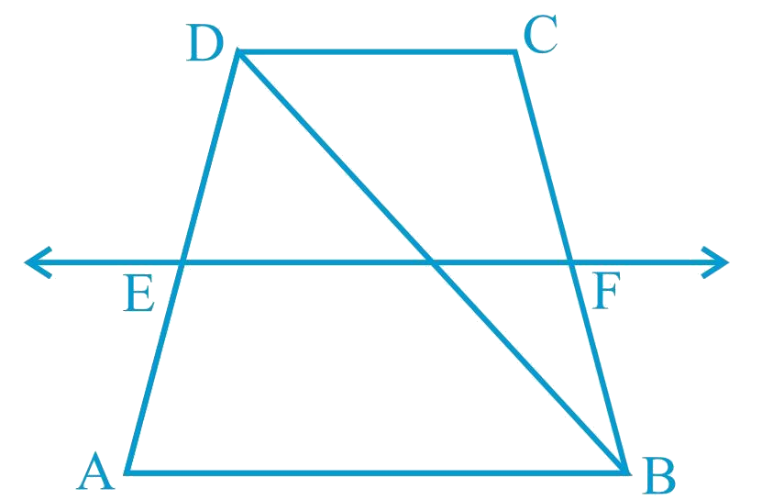### Solution

What is known?

ABCD is a trapezium in which $$AB DC, BD$$ is a diagonal and $$E$$ is the mid - point of $$AD.$$ A line is drawn through $$E$$ parallel to $$AB$$ intersecting $$BC$$ at $$F.$$

What is unknown?

How we can show that F is the mid-point of $$BC.$$

Reasoning:

By converse of mid-point theorem, we know that a line drawn through the mid-point of any side of a triangle and parallel to another side, bisects the third side.

Steps:

Let $$EF$$ intersect $$DB$$ at $$G$$.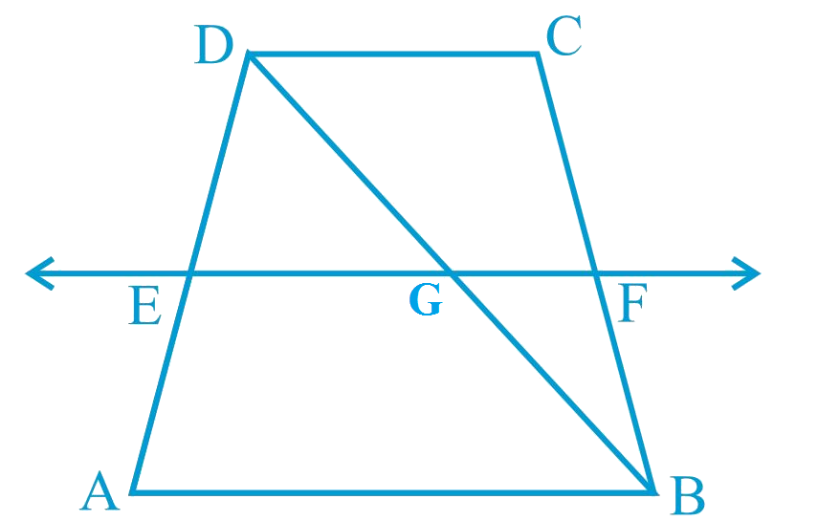By converse of mid-point theorem, we know that a line drawn through the mid-point of any side of a triangle and parallel to another side, bisects the third side.

In $$\Delta {ABD,}$$

$$EF$$ $$\parallel$$ $$AB$$ and $$E$$ is the mid-point of $$AD$$.

Therefore, $$G$$ will be the mid-point of $$DB$$.

As  $$EF$$ $$\parallel$$ $$AB$$ and $$AB$$ $$\parallel$$ $$CD$$,

$$\therefore$$  $$EF$$ $$\parallel$$ $$CD$$ (Two lines parallel to the same line are parallel to each other)

In $$\Delta {BCD,}$$ $$GF$$ $$\parallel$$ $$CD$$ and $$G$$ is the mid-point of line $$BD$$. Therefore, by using converse of mid-point theorem, $$F$$ is the mid-point of $$BC$$.

## Chapter 8 Ex.8.2 Question 5

In a parallelogram $$ABCD$$, $$E$$ and $$F$$ are the mid-points of sides $$AB$$ and $$CD$$ respectively (see the given figure). Show that the line segments $$AF$$ and $$EC$$ trisect the diagonal $$BD$$.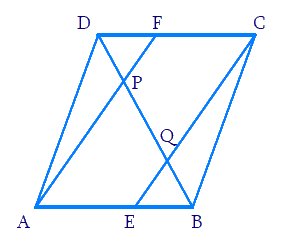### Solution

What is known?

In a parallelogram $$ABCD$$, $$E$$ and $$F$$ are the mid-points of sides $$AB$$ and $$CD$$ respectively.

What is unknown?

How we can show that the line segments $$AF$$ and $$EC$$ trisect the diagonal $$BD.$$

Reasoning:

In a quadrilateral if one pair of opposite sides is parallel and equal to each other. Then it is a parallelogram. Also by converse of mid-point theorem, we know that a line drawn through the mid-point of any side of a triangle and parallel to another side, bisects the third side.

Steps:

$$ABCD$$ is a parallelogram.

$$AB$$ $$\parallel$$ $$CD$$

And hence, $$AE$$ $$\parallel$$ $$FC$$

Again, $$AB = CD$$ (Opposite sides of parallelogram $$ABCD$$)

\begin{align}\frac{1}{2}{AB}=\frac{1}{2}{CD}\end{align}

$$AE = FC$$ ($$E$$ and $$F$$ are mid-points of side $$AB$$ and $$CD$$)

In quadrilateral $$AECF$$, one pair of opposite sides ($$AE$$ and $$CF$$) is parallel and equal to each other. Therefore, $$AECF$$ is a parallelogram.

$$\therefore$$  $$AF$$ $$\parallel$$ $$EC$$ (Opposite sides of a parallelogram)

In $$\rm \Delta DQC,$$ $$F$$ is the mid-point of side $$DC$$ and $$FP$$ $$\parallel$$ $$CQ$$ (as $$AF$$ $$\parallel$$ $$EC$$). Therefore, by using the converse of mid-point theorem, it can be said that $$P$$ is the mid-point of $$DQ$$.

$$\therefore$$ $$DP = PQ$$... (1)

Similarly, in $$\rm \Delta APB,$$ $$E$$ is the mid-point of side $$AB$$ and $$EQ$$ $$\parallel$$ $$AP$$ (as $$AF$$ $$\parallel$$ $$EC$$).

Therefore, by using the converse of mid-point theorem, it can be said that $$Q$$ is the mid-point of $$PB$$.

$$\therefore$$ $$PQ = QB$$ ... (2)

From Equations (1) and (2),

$$DP = PQ = BQ$$

Hence, the line segments $$AF$$ and $$EC$$ trisect the diagonal $$BD$$.

## Chapter 8 Ex.8.2 Question 6

Show that the line segments joining the mid-points of the opposite sides of a quadrilateral bisect each other.

### Solution

What is known?

$$ABCD$$ is a quadrilateral in which $$P, Q, R,$$ and $$S$$ are the mid-points of sides $$AB, BC, CD,$$ and $$DA$$ respectively.

What is unknown?

How we can show that the line segments joining the mid-points of the opposite sides of a quadrilateral bisect each other.

Reasoning:

In a triangle, the line segment joining the mid-points of any two sides of the triangle is parallel to the third side and is half of it. In a quadrilateral if one pair of opposite sides is parallel and equal to each other then it is a parallelogram.

Steps: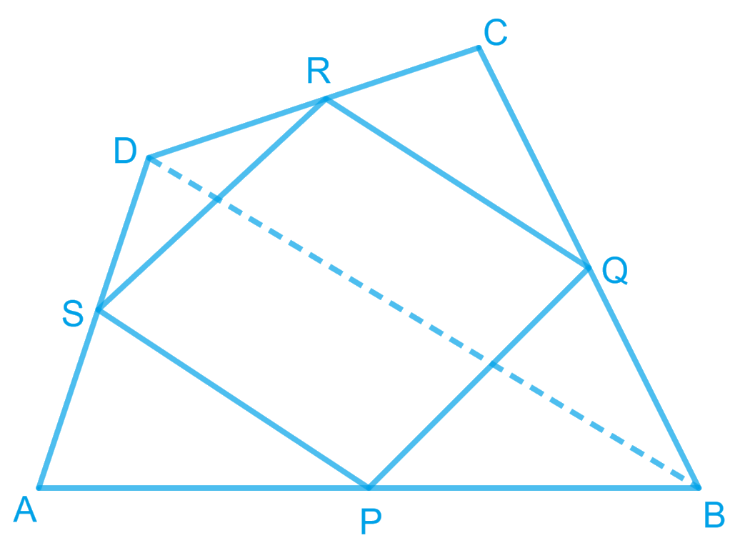Let $$ABCD$$ is a quadrilateral in which $$P$$, $$Q$$, $$R$$, and $$S$$ are the mid-points of sides $$AB$$, $$BC$$, $$CD$$, and $$DA$$ respectively. Join $$PQ$$, $$QR$$, $$RS$$, $$SP$$, and $$BD$$.

In $$\Delta {ABD,}$$ $$S$$ and $$P$$ are the mid-points of $$AD$$ and $$AB$$ respectively. Therefore, by using mid-point theorem, it can be said that

\begin{align} SP \parallel BD \; and\; SP\!=\!\frac{1}{2} BD \quad\!\!... (1) \end{align}

Similarly, in $$\Delta$$ $$BCD$$,

\begin{align} QR \parallel BD \; and\; QR \!=\!\frac{1}{2} BD \quad\!\!... (2) \end{align}

From Equations (1) and (2), we obtain

$$SP$$ $$\parallel$$$$QR$$ and $$SP = QR$$

In quadrilateral $$SPQR$$, one pair of opposite sides is equal and parallel to each other. Therefore, $$SPQR$$ is a parallelogram.

We know that diagonals of a parallelogram bisect each other.

Hence, $$PR$$ and $$QS$$ bisect each other.

## Chapter 8 Ex.8.2 Question 7

$$ABC$$ is a triangle right angled at $$C$$. $$A$$ line through the mid-point $$M$$ of hypotenuse $$AB$$ and parallel to $$BC$$ intersects $$AC$$ at $$D$$. Show that

(i) $$D$$ is the mid-point of $$AC$$

(ii) $$\rm{MD} \perp \rm{AC}$$

(iii)  \begin{align} \rm{CM}=\rm{MA}=\frac{1}{2} \rm{AB} \end{align}

### Solution

What is known?

$$ABC$$ is a triangle right angled at $$C$$. A line through the mid-point M of hypotenuse $$AB$$ and parallel to $$BC$$ intersects $$AC$$ at $$D.$$

What is unknown?

How we can show that

(i) $$D$$ is the mid-point of $$AC$$

(ii) $${MD }\!\!~\!\!\bot {AC}$$

(iii) $${CM}={MA}=\frac{1}{2}{ AB}$$

Reasoning:

By converse of mid-point theorem, we know that a line drawn through the mid-point of any side of a triangle and parallel to another side, bisects the third side. To show $$MD$$ perpendicular to $$AC$$ we know that sum of interior angles is $$180$$. Also to get $$CM=MA=$$half of AB we can show two triangles congruent

Steps: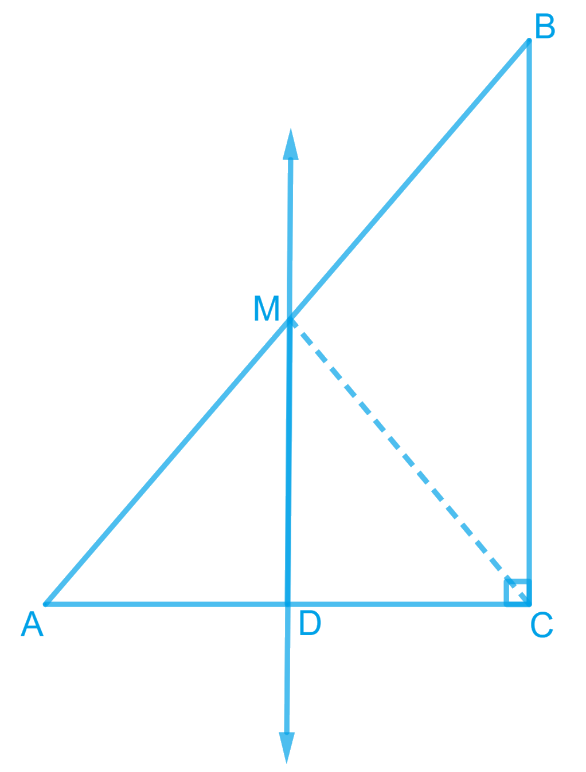(i) In $$\Delta {ABC,}$$

It is given that $$M$$ is the mid-point of $$AB$$ and $$MD$$ $$\parallel$$ $$BC$$.

Therefore, $$D$$ is the mid-point of $$AC$$. (Converse of mid-point theorem)

(ii) As $$DM$$ $$\parallel$$ $$CB$$ and $$AC$$ is a transversal line for them, therefore,

\begin{align} &\angle {MDC}+\angle {DCB}=180^{\circ}\\&({Co} \text { -interior angles })\\ &\angle {MDC}+90^{\circ}=180^{\circ}\\ &\angle {MDC}=90^{\circ}\\ &\therefore {MD} \perp {AC}\end{align}

(iii) Join $$MC$$.

In $$\Delta {AMD}$$ and $$\Delta {CMD}$$

$$AD = CD$$ (D is the mid-point of side $$AC$$)

\begin{align} \angle ADM= \angle CDM \; (Each\; 90^0 ) \end{align}

$$DM = DM$$ (Common)

\begin{align} \therefore \Delta {AMD}\cong \Delta {CMD}\end{align} (By SAS congruence rule)

Therefore, $$AM = CM$$ (By CPCT)

However, \begin{align} AM = \frac{1}{2} AB \end{align} (M is the mid-point of AB)

Therefore, it can be said that

\begin{align} CM= AM = \frac{1}{2} AB \end{align}

Related Sections
Related Sections
Instant doubt clearing with Cuemath Advanced Math Program